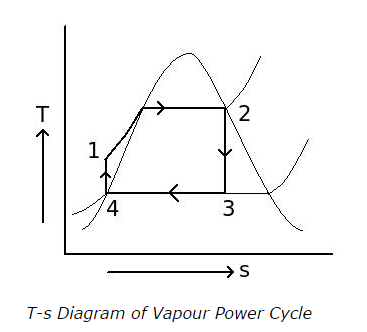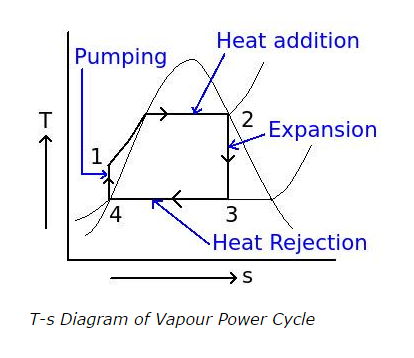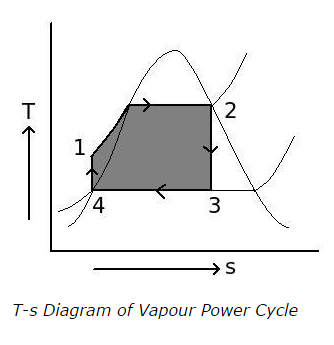# Rankine Cycle - MCQs with Answers

## Rankine Cycle - MCQs with Answers

Q1. Which processes do the Rankine cycle contain?

a. two isothermal and two isochoric processes
b. two isentropic and two isobaric processes
c. two isentropic and two isothermal processes
d. two isothermal and two isobaric processes

ANSWER: b. two isentropic and two isobaric processes

Q2. At ideal condition of vapour power cycle, reversible constant pressure heat rejection is carried out at

a. boiler
b. turbine
c. condenser
d. feed pump

Q3. Which ideal process is carried out at the turbine in vapour power cycle?

c. reversible constant pressure heat addition
d. reversible constant pressure heat rejection

Q4. In the T-s diagram of vapour power cycle, what is the condition of stem at the starting of turbine expansion? (point 2)a. wet with dryness fraction 0.8
b. wet with dryness fraction 0.99
c. dry saturated
d. superheated

Q5. In the following T-s diagram of Rankine cycle, process (1-2) is heat addition in boiler, process (2-3) is an expansion at turbine, process (3-4) is a condensation process and process (4-1) is a pumping process at feed pump. What is the formula for efficiency of Rankine cycle?a. ηRankine = ((h2 – h3) + (h1 – h4)) / (h2 – h1)
b. ηRankine = ((h2 – h3) – (h1 – h4)) / (h2 – h1)
c. ηRankine = (h2 – h3) / (h2 – h1)
d. none of the above

ANSWER: b. ηRankine = ((h2 – h3) – (h1 – h4)) / (h2 – h1)

Q6. Sometimes the pump work in vapour power cycle is neglected because

a. the pump work in not considered in efficiency of vapour power cycle
b. the pump work is very small compared to the heat addition
c. the pump work is very small compared to the turbine work
d. none of the above

ANSWER: b. the pump work is very small compared to the heat addition

Q7. How is the capacity of vapour power plant expressed?

a. in terms of heat rate
b. in terms of steam rate
c. in terms of work output
d. none of the above

ANSWER: b. in terms of steam rate

Q8. What is heat rate in steam power plant?

a. the rate of heat input in kJ per heat input in kW
b. the rate of heat input in kJ required to produce unit turbine work (1 kW)
c. the rate of heat input in kJ required to produce unit net shaft work (1 kW)
d. none of the above

ANSWER: c. the rate of heat input in kJ required to produce unit net shaft work (1 kW)

Q9. What is the highlighted area (area enclosed by 1234) in the following T-s diagram of vapour power cycle indicate?b. work produced by turbine
c. net work produced by cycle
d. heat rejected from cycle

ANSWER: c. net work produced by cycle

Q10. To maximize the work output at turbine, the specific volume of working fluid should be

a. as small as possible
b. as large as possible
c. constant throughout the cycle
d. none of the above

ANSWER: b. as large as possible

Q11. For the same pressure ratio, what is the relation between work required to compress steam in vapour form and work required to compress steam in liquid form?

a. work required to compress steam in vapour form is equal work required to compress steam in liquid form

b. work required to compress steam in vapour form is more than work required to compress steam in liquid form

c. work required to compress steam in vapour form less than work required to compress steam in liquid form

d. cannot say

ANSWER: b. work required to compress steam in vapour form is more than work required to compress steam in liquid form

Q12. Which is the affecting factor for the fact that turbine work output is more than pump work input in vapour power cycle for the same pressure ration?

a. specific heat added to the working fluid
b. specific volume of working fluid
c. both a. and b.
d. none of the above